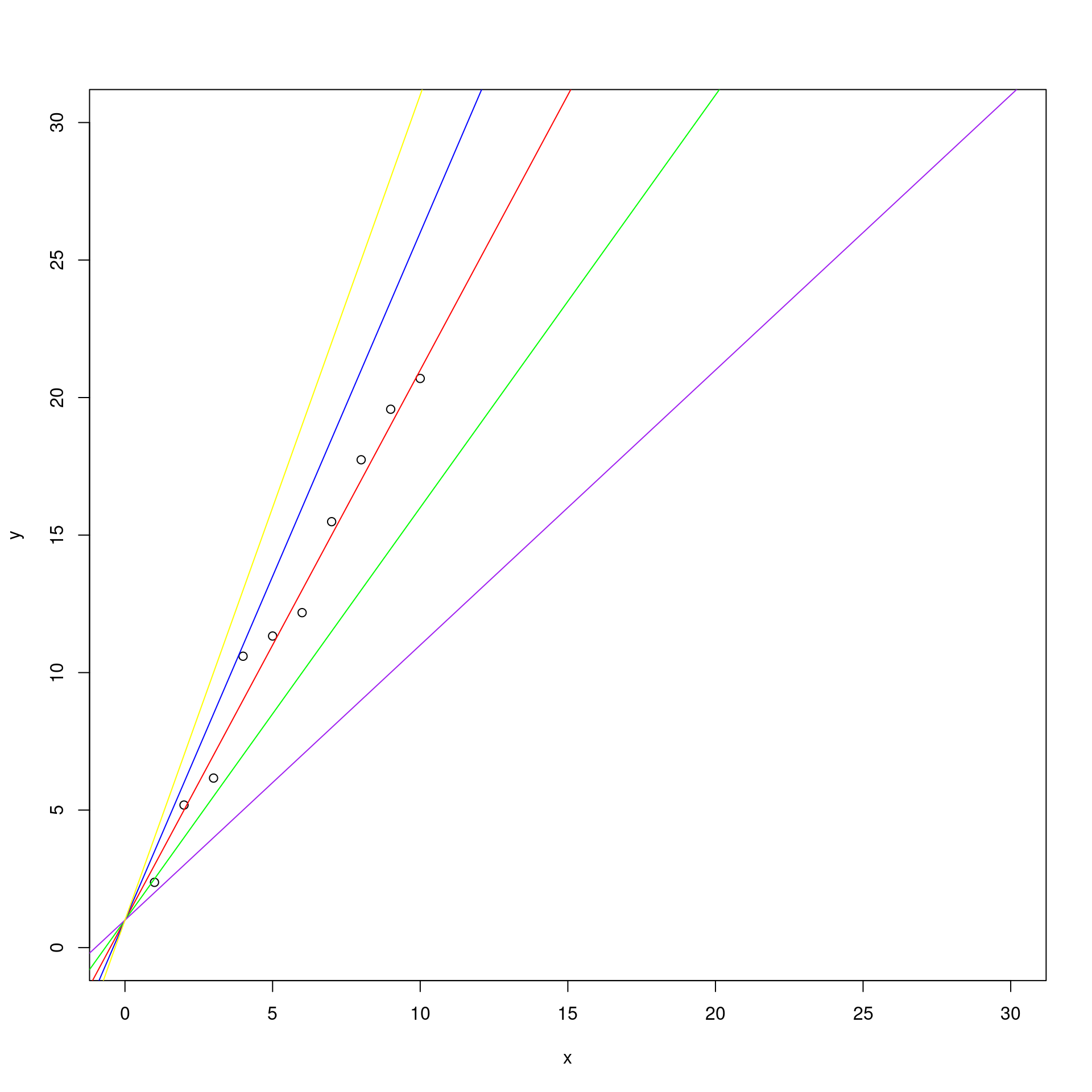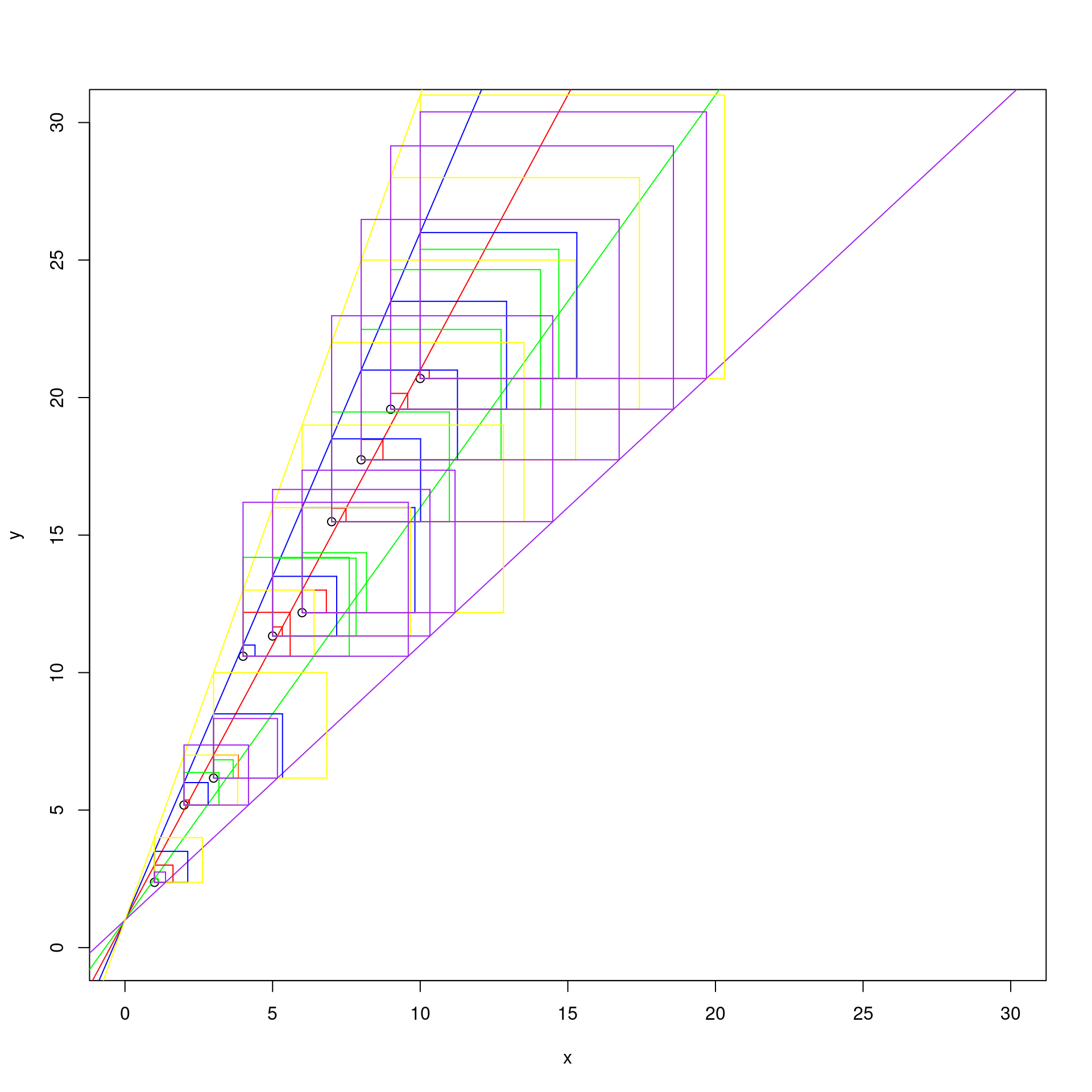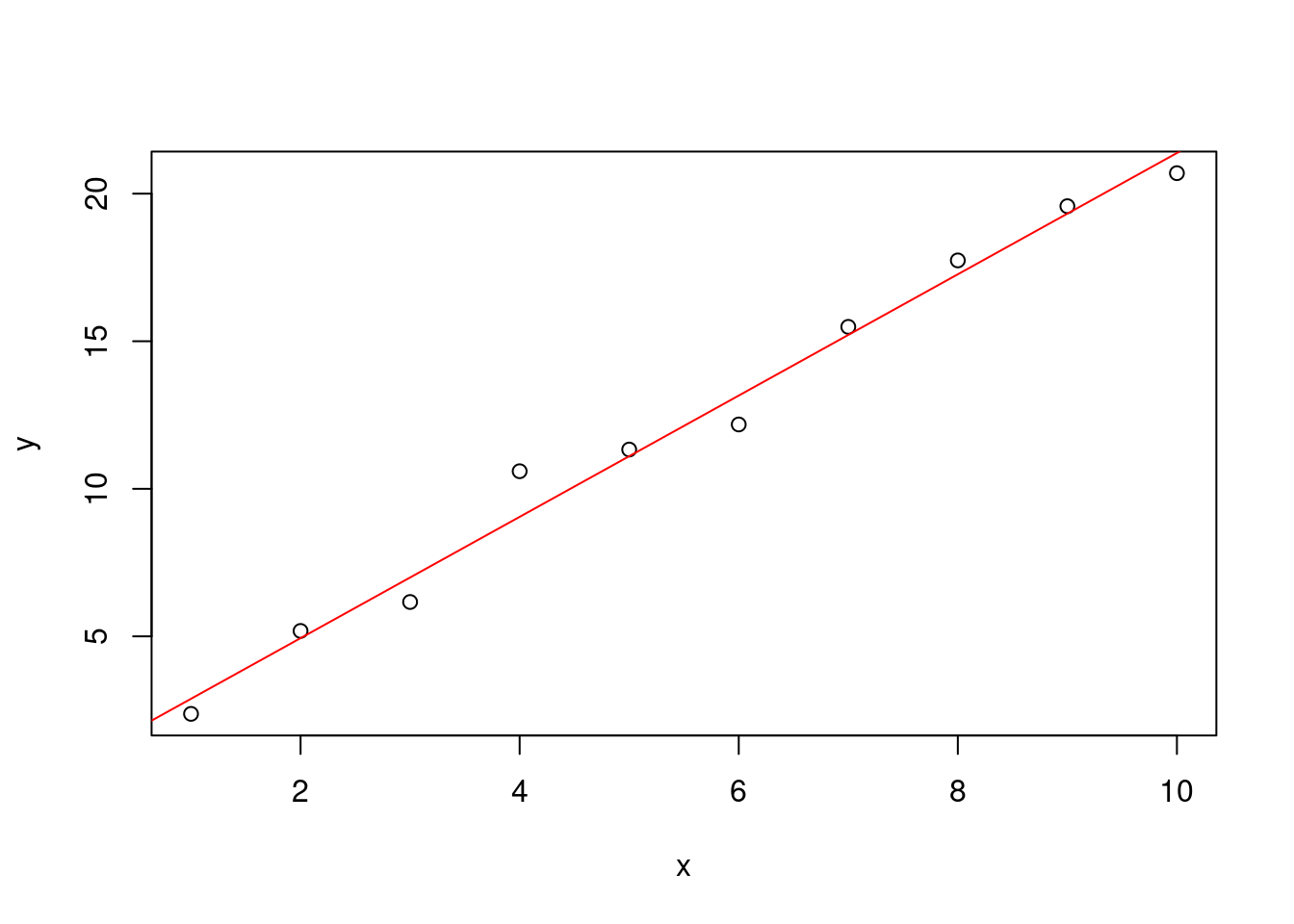# Linear regressions

true

Linear regression is not difficult, basically we are trying to estimate a line that efficiently interpolates our data.

However, how it works?

## Independent and Dependent variables

Linear regression try to estimate the parameters $$\beta$$ (independent variables) that allow to better “explain the variance” of observations (dependent variable).

The dependent variable is the observation that we postulate being shaped / modulated / caused by our independent variables.

This means that we are not looking for correlations between the dependent and independent variables, because we assume that the dependent depends (sorry…) from the independent variables. Converserly, correlations do not assume that a variable causes the modification of another variable. In fact, in the correlation case, it is an error speaking of dependent and independent variables.

## The linear regression formula

Therefore, we try to estimate the unknown vector of parameters $$\beta$$ in this formula $$y = X \beta + \epsilon$$ trying to minimize $$\epsilon$$, that is our error term.

$$\beta$$ could also be named coefficients, fixed effects, regressors, etc…

In linear models the error term $$\epsilon$$ should be distributed along a normal distribution with mean 0 and standard deviation 1 $$\epsilon \sim N(0, 1)$$.

The $$X$$ matrix represents the contrast matrix where all our independent data are stored: covariates and factors.

# Estimation of the unknown vector of $$\beta$$s

Usually the estimation of the $$\beta$$s can be computed with one ot hese three algorythms:

1. Ordinary Least Square estimation (OLS)
2. Maximum Likelihood estimation (ML)
3. Restricted (or Residual) Maximum Likelihood estimation (REML)

## Ordinary Least Square estimation (OLS)

The idea is to find the coefficients that describe the line whose difference between the fitted / predicted data (i.e. the estimation of the observed data that are obtained by using the fitted model) and the observed / dependent data is minimal.

Namely, $$\beta = \underset{\beta}{\operatorname{arg min}}~S(\beta)$$, where $$S(\beta) = \sum_{i=1}^{n} | y_i - \sum_{j=1}^p x_{i,j} \beta_{j}|^2$$ , that basically is the square of the error of the prediction between the observed ($$y$$) and the predicted ($$\hat{y}$$) data.

Therefore, among all the following lines:

## creating the data
set.seed( 1 )
# independent variable
x <- 1:10
#   beta0 + i.v. * beta1 + epsilon
y <- 1 + x * 2 + rnorm( n = 10 )

## plotting the data
plot( x , y , ylim = c(0, 30) , xlim = c (0, 30))
abline(a = 1, b = 2 , col = "red")
abline(a = 1, b = 1.5, col = "green")
abline(a = 1, b = 2.5, col = "blue")
abline(a = 1, b = 1, col = "purple")
abline(a = 1, b = 3, col = "yellow")OLS will choose the one with the smallest square of errors $$S(\beta)$$.

## creating the data
set.seed( 1 )
# independent variable
x <- 1:10
#   beta0 + i.v. * beta1 + epsilon
y <- 1 + x * 2 + rnorm( n = 10 )

## plotting the data
plot( x , y , ylim = c(0, 30) , xlim = c (0, 30))
abline(a = 1, b = 2 , col = "red")
abline(a = 1, b = 1.5, col = "green")
abline(a = 1, b = 2.5, col = "blue")
abline(a = 1, b = 1, col = "purple")
abline(a = 1, b = 3, col = "yellow")

d1 <- abs( y - (1 + x * 2) )
d2 <- abs( y - (1 + x * 1.5) )
d3 <- abs( y - (1 + x * 2.5) )
d4 <- abs( y - (1 + x * 3) )
d5 <- abs( y - (1 + x) )

segments(x0 = x, y0 = y, x1 = x, y1 = y + d1, col = "red")
segments(x0 = x, y0 = y, x1 = x + d1, y1 = y, col = "red")
segments(x0 = x + d1, y0 = y, x1 = x + d1, y1 = y + d1, col = "red")
segments(x0 = x, y0 = y + d1, x1 = x + d1, y1 = y + d1, col = "red")

segments(x0 = x, y0 = y, x1 = x, y1 = y + d2, col = "green")
segments(x0 = x, y0 = y, x1 = x + d2, y1 = y, col = "green")
segments(x0 = x + d2, y0 = y, x1 = x + d2, y1 = y + d2, col = "green")
segments(x0 = x, y0 = y + d2, x1 = x + d2, y1 = y + d2, col = "green")

segments(x0 = x, y0 = y, x1 = x, y1 = y + d3, col = "blue")
segments(x0 = x, y0 = y, x1 = x + d3, y1 = y, col = "blue")
segments(x0 = x + d3, y0 = y, x1 = x + d3, y1 = y + d3, col = "blue")
segments(x0 = x, y0 = y + d3, x1 = x + d3, y1 = y + d3, col = "blue")

segments(x0 = x, y0 = y, x1 = x, y1 = y + d4, col = "yellow")
segments(x0 = x, y0 = y, x1 = x + d4, y1 = y, col = "yellow")
segments(x0 = x + d4, y0 = y, x1 = x + d4, y1 = y + d4, col = "yellow")
segments(x0 = x, y0 = y + d4, x1 = x + d4, y1 = y + d4, col = "yellow")

segments(x0 = x, y0 = y, x1 = x, y1 = y + d5, col = "purple")
segments(x0 = x, y0 = y, x1 = x + d5, y1 = y, col = "purple")
segments(x0 = x + d5, y0 = y, x1 = x + d5, y1 = y + d5, col = "purple")
segments(x0 = x, y0 = y + d5, x1 = x + d5, y1 = y + d5, col = "purple")By observing the graph, we can say that is the red one, that has as coefficients $$\beta_0 = 1$$ and $$\beta_1 = 2$$.

In order to see if analytically is as we observed, we can start with an iterative inefficient function:

find_least_squares <- function( y , x , beta0 , beta1 ) {

# variable for the beta1 that best fits the data
best_beta <- c(NULL, NULL)
# variable for memorizing the squared errors
best_err  <- Inf
for(int in beta0){
for( bet in beta1 ){
yhat <- int + x * bet
err  <- sum( abs( y - yhat)^2 )
best_beta <- ifelse( best_err > err,
int,
best_beta)
best_beta <- ifelse( best_err > err,
bet,
best_beta)
best_err  <- ifelse( best_err > err,
err,
best_err)
}
}

return( best_beta )
}

print( find_least_squares( y , x, beta0 = seq(from = 0, to = 3, by = 0.1),
beta1 = seq(from = 1, to = 3, by = 0.1) ) )
##  0.6 2.1

Let’s try to estimate these values with the standard function of R lm (that does not uses OLS to estimate the coefficients [thanks Marco Tullio Liuzza for the correction]):

mdl <- lm(y ~ x)

print( mdl )
##
## Call:
## lm(formula = y ~ x)
##
## Coefficients:
## (Intercept)            x
##      0.8312       2.0547

As you can see, very similar same results.

plot( x , y )
abline(mdl , col= "red")## Maximum Likelihood estimation (ML)

In this case we want to maximize the probability of a Likelihood function in describing the data. Therefore, we want to find the values of $$\beta$$ that maximize the probability of describing the observed dependent variable $$y$$, namely the values of $$\beta$$ that, if use din our model, maximize the probability of obtaining the observed data.

Namely, $$\beta = \underset{\beta}{\operatorname{arg max}}~\hat{L}_n(\beta;y)$$, where $L_n(;y)$ is the Likelihood function, that is also an index of goodness of fit of the model (the log-likelihood is often used for model selection).

To better understand how it works, here another interactive, inefficient function (remember that we postulate that our coefficients are distributed along normal distributions):

my_gauss <- function(true_value, sigma, estimate) 1/(sigma*sqrt(2*pi)) *
exp(- (true_value - estimate)^2/(2*sigma^2))

Thanks to the above function, I will get the likelihood of each parameter, given its true value and the proposed estimate value.

We know that our data were crated with a angular coefficient of 2, therefore this is our true value. We will try different estimate values to see which has the higher likelihood.

find_ML <- function( beta0 , beta1 ) {

# variable for the beta1 that best fits the data
best_betas <- c(NULL , NULL)
# variable for memorizing the squared errors
best_lik  <- -Inf

for(int in beta0){
for( bet in beta1 ){
current_lik <- my_gauss(1 , sigma = 1 , int) *
my_gauss(2 , sigma = 1 , bet)

best_betas <- ifelse( current_lik > best_lik,
int,
best_betas)

best_betas <- ifelse( current_lik > best_lik,
bet,
best_betas)

best_lik  <- ifelse( current_lik > best_lik,
current_lik,
best_lik)
}
}

return( best_betas )
}

print( find_ML( beta0 = seq(from = 0, to = 3, by = 0.1),
beta1 = seq(from = 1, to = 3, by = 0.1) ) )
##  1 2

As we can see, also in this case the results are 1 for the intercept and 2 for the angular coefficient.

This methodology can be used in both linear and multilevel linear models.

## Restricted (or Residual) Maximum Likelihood (REML)

This method is used in particular in linear models that take into consideration both population- and group-level effects.

As in ML, we want to maximize the Likelihood of the model by finding the best $$\beta$$s parameters. Remember that in linear models all $$\beta$$s are distributed along a normal distribution characterized by a $$\mu$$ and $$\sigma$$ parameter:

$$\beta \sim N(\mu,\sigma)$$

With this methodology, the computation of the Likelihood of the model is composed by two parts, maximised separately:

1. The Likelihood of $$\mu$$ and $$\sigma$$ parameters for all group- and population-level effects. $$\leftarrow$$ to maximize the likelihood of the $$\mu$$ parameter.
2. The Residual Likelihood of only the $$\sigma$$ parameters. $$\leftarrow$$ to maximize the likelihood of the $$\sigma$$ parameter.